$$\require{cancel}$$

# B18: Faraday's Law and Lenz's Law

Do you remember Archimedes’s Principle? We were able to say something simple, specific, and useful about a complicated phenomenon. The gross idea was that a submerged object being pressed upon on every surface element in contact with fluid, by the fluid, experiences a net upward force because the pressure in a fluid is greater at greater depth. The infinite sum, over all the surface area elements of the object in contact with the fluid, of the force of magnitude pressure-times-the area, and direction normal to and into the area element, resulted in an upward force that we called the buoyant force. The thing is, we were able to prove that the buoyant force is equal in magnitude to the weight of that amount of fluid that would be where the object is if the object wasn’t there. Thus we can arrive at a value for the buoyant force without having to even think about the vector integration of pressure-related force that causes it.

We are about to encounter another complicated phenomenon which can be characterized in a fruitful fashion by a relatively simple rule. I’m going to convey the idea to you by means of a few specific processes, and then sum it up by stating the simple rule.

Consider a gold ring and a bar magnet in the hands of a person. The person is holding the ring so that it encircles the bar magnet. She is holding the magnet, north end up.There is a magnetic field, due to the bar magnet, within the bar magnet, and in the region of space around it.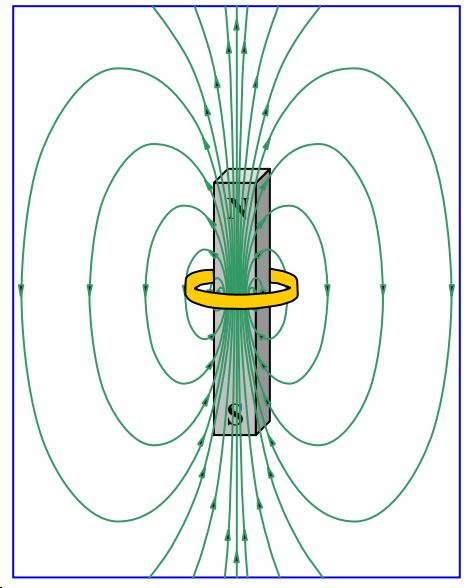It is important to note that the magnetic field lines are most densely packed inside the bar magnet.

Now suppose that the person, holding the magnet at rest in one hand, moves the loop upward. I want to focus on what is going on while she is moving it upward. As she moves the loop upward, she is moving it roughly along the direction of the magnetic file lines, but, and this is actually the important part, that loop will also be “cutting through” some magnetic field lines. Consider an instant in time when the loop is above the magnet, and moving upward: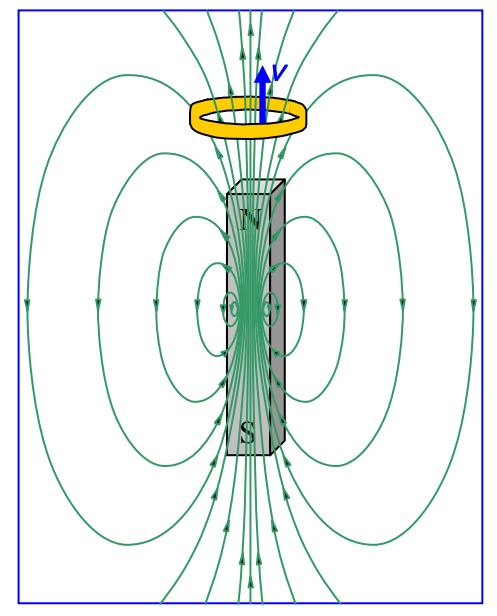From above, the scene looks like:where it is important to realize that none of those magnetic field lines begin on the magnet or end at the tip of the arrow depicted, rather, they extend out of the magnet toward us, flower out and over, back down away from us, and then they loop around to enter the south pole of the magnet from which they extend back up through the magnet toward us. In fact, no magnetic field line ever begins or ends anywhere. They all form closed loops. This is a manifestation of the fact that there is no such thing as magnetic charge. (There are no magnetic monopoles.)Here’s where we’re going with this: The motion of the ring relative to the magnet is going to cause a current in the ring. Here’s how: The ring is neutral, but, it is chock full of charged particles that are free to move around within the gold. [I’m going to discuss it in our positive charge carrier model but you can verify that you get the same result if the charge carriers are negative (recalling that our current is in the direction opposite that in which negative charge carriers are moving.)] Pick any short segment of the ring and get the direction of the force exerted on the charge carriers of that segment using $$\vec{F}=q\vec{v}\times \vec{B}$$ and the right-hand rule for the cross product of two vectors. In the view from above, all we can see is the horizontal component of the magnetic field vectors in the vicinity of the moving ring but that’s just dandy; the vertical component, being parallel to the ring’s velocity (and hence parallel to the velocity of the charge in the ring), makes no contribution to $$\vec{v}\times \vec{B}$$. Now, pick your segment of the ring. Make your fingers point away from your elbow, and, in the direction of the first vector (the velocity vector) in $$\vec{v}\times \vec{B}$$, namely, “out of the page”. Now, keeping your fingers pointing both away from your elbow, and, out of the page, rotate your forearm as necessary so that your palm is facing in the direction of $$\vec{B}$$ (at the location of the segment you are working on), meaning that if you were to close your fingers, they would point in the direction of $$\vec{B}$$. Your extended thumb is now pointing in the direction of the force exerted on the positive charge carriers in the ring segment you chose. No matter what ring segment you pick, the force is always in that direction which tends to push the positive charge carriers counterclockwise around the ring! The result is a counterclockwise (as viewed from above) current in the ring.

Suppose that, starting with the ring encircling the magnet, the person who was holding the ring and the magnet moved the magnet downward rather than moving the ring upward. She holds the ring stationary, and moves the magnet. I said earlier that a charged particle at rest in a magnetic field has no force exerted on it by the magnetic field. But we were talking about stationary magnetic fields at the time. Now we are talking about the magnetic field of a magnet that is moving. Since the magnet responsible for it is moving, the magnetic field itself must be moving. Will that result in a force on the charges in the ring (and hence a current in the ring)? This brings us to a consideration of relative motion. To us, the two processes (person moves ring upward while holding magnet at rest, vs. person moves magnet downward while holding ring at rest) are different. But that is just because we are so used to viewing things from the earth’s reference frame. Have you ever been riding along a highway and had the sense that you were at rest and the lampposts on the side of the road were moving past you at high speed. That is a valid viewpoint. Relative to your reference frame of the car, the lampposts are indeed moving and the car is a valid reference frame. Suppose we view the magnet moving downward through a ring situation from a platform that is moving downward at the same speed as the magnet. In that reference frame, the magnet is at rest. If for instance, as we, while seated on the platform, see the magnet at eye level, it remains at eye level. But the ring, as viewed from the platform reference frame is moving upward. So in the platform reference frame, we have, in the new processes (which in the room reference frame is a magnet moving downward through and away from a ring) the same situation that we had in the room frame for the original process (which in the room reference frame is a ring, originally encircling a stationary magnet, moving upward). Thus in the platform reference frame, we must have the same result for the new process that we had for the original process in the room frame, namely, a counterclockwise (as viewed from above) current in the ring. The current in the ring doesn’t depend on what reference frame we view the ring from. Hence, we can conclude that the magnet moving downward through the stationary ring at speed $$v$$ results in the same current as we have when the ring moves upward at the same speed $$v$$ relative to the stationary magnet.

When the person holding the magnet and the ring moved the ring upward, there was a current in the ring. Now we have established that if, instead of moving the ring, she moves the magnet downward at the same speed, she will get the same current in the ring. Based on what caused that current, the $$\vec{F}=q\vec{v}\times \vec{B}$$ force on the charged particles in the ring, you can surmise that the current will depend on things like the velocity of the ring relative to the magnet, the strength of the magnetic field, and the relative orientation of the velocity vector and the magnetic field. It has probably occurred to you that the current also depends on the resistance of the ring.

Michael Faraday came up with a very fruitful way of looking at the phenomenon we are discussing and I will convey his idea to you by means of the example we have been working with.

Looking at the diagrams of that ring moving relative to the magnet again,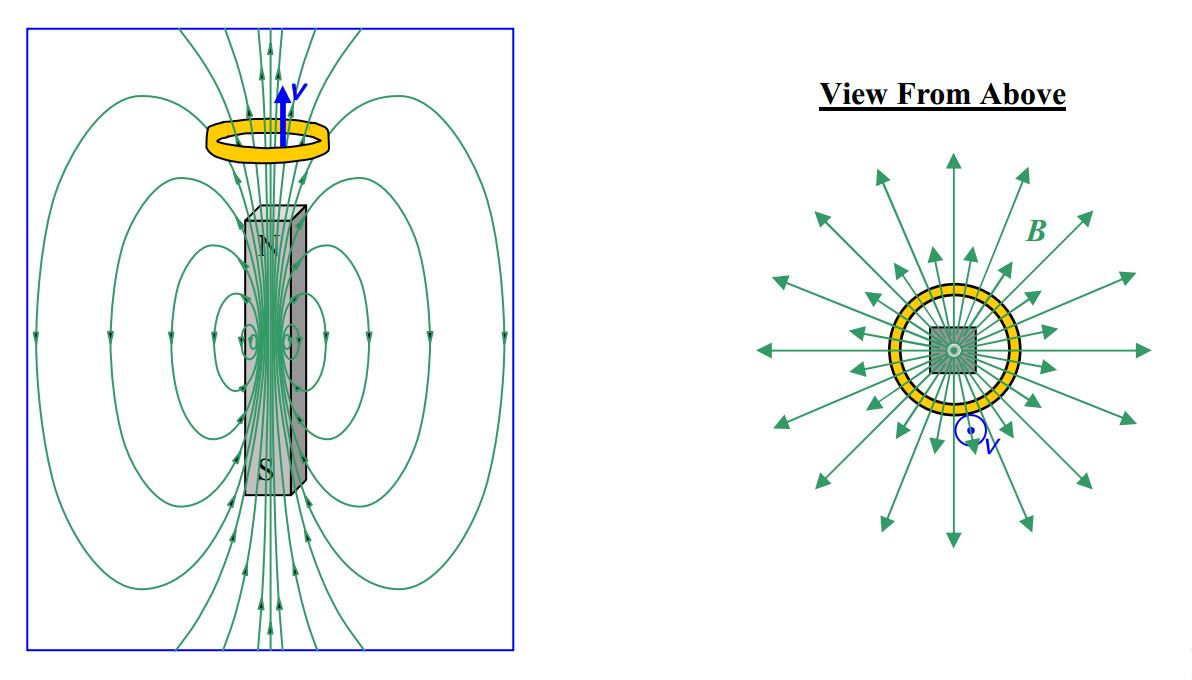we can describe what’s happening by saying that the ring is “cutting through” magnetic field lines (or, equivalently, by saying that the magnetic field lines are “cutting through” the ring). What Faraday recognized was that, in conceptual terms, by the ring cutting through magnetic field lines (or vice versa depending on your point of view), what was happening was, that the number of magnetic field lines encircled by the loop was changing. In the diagrams above, each time the ring “cuts through” one more field line, the number of field lines encircled by the loop decreases by one. The rate at which the ring “cuts through” magnetic field lines (or the magnetic field lines cut through the ring) is determined by the same things that determine the force on the charged particles making up the ring (relative speed between ring and magnetic field, strength of magnetic field, relative orientation of velocity of ring and magnetic field) such that, the greater the rate at which the ring “cuts through” magnetic field lines (or the greater the rate at which magnetic field lines cut through the ring), the greater the force on the charged particles and hence the greater the current. Faraday expressed this in a manner that is easier to analyze. He said that the current is determined by the rate at which the number of magnetic field lines encircled by the loop is changing. In fact, Faraday was able to write this statement in equation form. Before I show you that, I have to be a lot more specific about what I mean by “the number of magnetic field lines.”

I’m going to call the statement I have just attributed to Faraday, the conceptual form of Faraday’s Law. In other words, Faradays Law, in conceptual form is: A changing number of magnetic field lines through a closed loop or coil causes a current in that loop or coil, and, the faster the number is changing, the greater the current.

Our field line concept is essentially a diagrammatic scheme used to convey some information about the direction and the relative strength of a field. We have used it both for the electric field and the magnetic field. What I say here about the number of field lines can be applied to both, but, since we are presently concerned with the magnetic field, I will talk about it in terms of the magnetic field. Conceptually, the number of field lines encircled by a loop is going to depend on how closely packed the field lines are, how big the loop is, and to what degree the loop is oriented “face-on” to the field lines. (Clearly, if the loop is oriented edge-on to the field lines, it will encircle none of them.) Now, diagrammatically, how closely packed the field lines are is representative of how strong the magnetic field is. The more closely-packed the field lines, the greater the value of B. Imagine that someone has created a beautiful, three-dimensional, magnetic field diagram. Now if you view the field lines end-on, e.g. such that the magnetic field lines are directed right at you, and depict a cross section of “what you see” in a two-dimensional diagram, you would get something like this.This is a graphical representation of the magnitude of that component of the magnetic field which is directed straight at you.

Suppose the scale of the diagram to be given by ($$1\mu T\cdot m^2$$)n where n is the magnetic field line density, the number-of-magnetic-field-lines-per-area, directed through the plane represented by the page, straight at you. Let’s use a square, one centimeter on a side, to sample the field at a position near the center,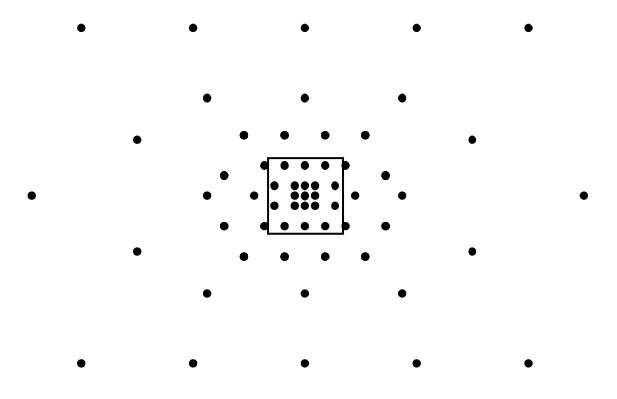I count $$19$$ field lines that are clearly in the square centimeter and four that are touching it, I’m going to count two of those four for an estimated $$21$$ field lines in one square centimeter. Thus, in that region,

$n=\frac{21 \, \mbox{lines}}{(1\times 10^{-2}m)^2}$

$n=2100\frac{\mbox{lines}}{\mbox{m}^2}$

Using the given scale factor,

$B=(1.0\mu T\cdot m^2)n$

$B=(1.0\mu T\cdot m^2) 2100\frac{\mbox{lines}}{\mbox{m}^2}$

$B=2.1 mT$

Let’s make it more clear what the number of lines represents by replacing $$n$$ with $$\frac{\mbox{Number of Lines}}{A}$$ and solving the expression $$V=(1.0\mu T)n$$ for the number of lines.

$B=(1.0\mu T\cdot m^2)\frac{\mbox{Number of Lines}}{A}$

$\mbox{Number of Lines}=\frac{BA}{1.0\mu T\cdot m^2}$

So the number of lines through a loop encircling a plane region of area $$A$$ is proportional to $$BA$$, with the constant of proportionality being the reciprocal of our scale factor for the field diagram. The simple product $$BA$$ is really only good if the magnetic field lines are “hitting” the area encircled by the loop “head on,” and, if the magnetic field is single-valued over the whole area. We can take care of the “which way the loop is facing” issue by replacing $$BA$$ with $$\vec{B}\cdot \vec{A}$$ where $$\vec{A}$$, the area vector, is a vector whose magnitude is the area of the plane region encircled by the loop and whose direction is perpendicular to the plane of the loop. There are actually two directions that are perpendicular to the loop. One is the opposite of the other. In practice, one picks one of the two directions arbitrarily, but, picking a direction for the area vector establishes a positive direction for the current around the loop. The positive direction for the current is the direction around the loop that makes the current direction and the area vector direction, together, conform to the right-hand rule for something curly something straight. We take care of the possible variation of the magnetic field over the region enclosed by the loop, by cutting that plane region up into an infinite number of infinitesimal area elements $$dA$$, calculating $$\vec{B}\cdot \vec{dA}$$ for each area element, and adding up all the results. The final result is the integral $$\int \vec{B}\cdot \vec{dA}$$. You won’t be held responsible for using the calculus algorithms for analyzing such an integral, but, you are responsible for knowing what $$\int \vec{B}\cdot \vec{dA}$$ means. It is the infinite sum you get when you subdivide the area enclosed by the loop up into an infinite number of infinitesimal area elements, and, for each area element, dot the magnetic field vector at the location of that area element into the area vector of that area element, and add up all the resulting dot products. You also need to know that, in the special case of a magnetic field that is constant in both magnitude and direction over the entire area enclosed by the loop, $$\int \vec{B}\cdot \vec{dA}$$ is just $$\vec{B}\cdot \vec{A}$$.

Using a generic “constant” for the reciprocal of the field diagram scale factor yields

$\mbox{Number of Lines}=(constant) \int \vec{B}\cdot \vec{dA}$

for the number of field lines encircled by the loop or coil. The quantity $$\int \vec{B}\cdot \vec{dA}$$ is called the magnetic flux through the plane region enclosed by the loop. Note that the flux is directly proportional to the number of magnetic field lines through the loop.

The magnetic flux is given the name $$\Phi_B$$ (the Greek letter upper case phi).

$\Phi_B=\int \vec{B}\cdot \vec{dA}$

The expression yields $$T\cdot m^2$$ as the units of magnetic flux. This combination of units is given a name, the Weber, abbreviated Wb.

$1Wb=T\cdot m^2$

Faraday’s Law, the one that says that the current induced in a loop or coil is proportional to the rate of change in the number of magnetic field lines encircled by the loop or coil, can be written in terms of the flux as:

$I=-\frac{N}{R} \frac{d\Phi_B}{dt}$

where:

$$N$$ is the number of windings or turns making up the closed coil of wire. $$N =1$$ for a single loop.

$$R$$ is the resistance of the loop or coil.

$$\frac{d\Phi_B}{dt}$$ is the rate of change in the flux through the loop.

The derivative of a function with respect to time is often abbreviated as the function itself with a dot over it. In other words,

$\dot{\Phi_B}=\frac{d\Phi_B}{dt}$

Using this notation in our expression for the current in Faradays Law of induction we have:

$I=-\frac{N}{R} \dot{\Phi_B}$

Faraday’s Law is usually expressed in terms of an EMF rather than a current. I’m going to use a specific case study to develop the idea which is of general applicability. Consider a coil of ideally-conducting wire in series with a resistor. For closure of the loop, the resistor is to be considered part of the loop (and hence is the resistance of the loop), but, we have a negligible number of magnetic field lines cutting through the resistor itself. Suppose there to be an increasing magnetic flux directed upward through the coil.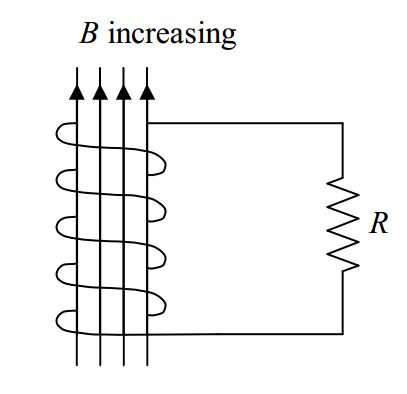By Faraday’s Law of Induction, there will be a current $$I=-\frac{N}{R} \dot{\Phi_B}$$ induced in the coil. The charge will flow around and around the coil, out the top of the coil and down through the resistor.But, for a resistor to have a current in it, there must be a potential difference $$V=IR$$ between the terminals of the resistor.Recognizing that, in the case at hand, the $$I$$ in $$V = IR$$ is the $$I=-\frac{N}{R} \dot{\Phi}_B$$ resulting from the changing magnetic flux through the coil, we have

$V=\Big( -\frac{N}{R}\dot{\Phi}_B \Big) R$

which we can write as

$V=-N\dot{\phi}_B$

Where there is a voltage across a resistor, there is an electric field in the resistor. What exactly causes that electric field? The answer is, the changing flux through the coil. More specifically, it is the magnetic field lines cutting through the coil as they must be doing to cause a change in the number of field lines through the coil. The field lines through the coil causes a force on the charge carriers in the coil. In our positive charge carrier model, this causes positive charge carriers in the coil all to surge toward the top of the resistor, leaving an absence of same on the bottom of the resistor. It only takes a minuscule amount of charge to cause an appreciable electric field in the resistor. A dynamic equilibrium is reached in which the changing magnetic field force on the charged particle becomes unable to push any more charge to the top terminal of the resistor than is forced through the resistor by the electric field in the resistor. The changing magnetic field can’t push more charge there because of the repulsion of the charge that is already there. The changing magnetic field force in the coil maintains the potential difference across the resistor in spite of the fact that charge carriers keep “falling” through the resistor. This should sound familiar. A seat of EMF does the same thing. It maintains a constant potential difference between two conductors (such as the terminals of the resistor in the case at hand). The coil with the changing flux through it is acting like a seat of EMF. One says that the changing flux induces an EMF in the coil, calls that Faraday’s Law of Induction, and writes:

$\varepsilon=-N \dot {\Phi}_B$

where:

• $$\varepsilon$$ is the EMF induced in the loop.
• $$N$$ is the number of windings or turns making up the coil of wire.
• $$\dot{\Phi}_B$$ is the rate of change in the flux through the loop.